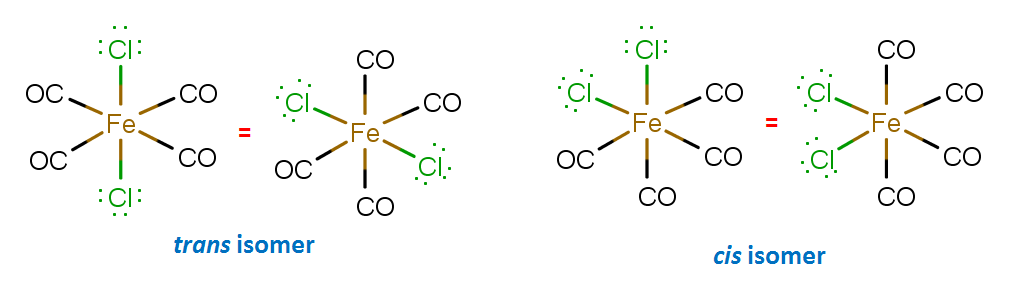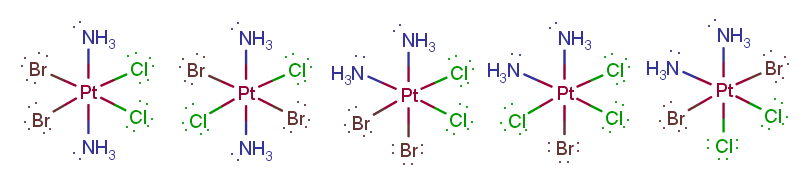# Problem: 1. How many geometric isomers are there for each species?[Fe(CO)4Cl2]    [Pt(NH3)2Cl2Br2]+2. How many unpaired electrons would you expect for the complex ion: [FeCl6]4-?3. What type of geometry (according to valence bond theory) does Fe exhibit in the complex ion, [Fe(H2O)4(Br)2]+?a) tetrahedralb) linearc) quare planard) octahedrale) trigonal planar

###### FREE Expert Solution

1. How many geometric isomers are there for each species?

[Fe(CO)4Cl2]2 geometrical isomers

[Pt(NH3)2Cl2Br2]+5 geometrical isomers

2. How many unpaired electrons would you expect for the complex ion: [FeCl6]4-?

82% (216 ratings)###### Problem Details

1. How many geometric isomers are there for each species?

[Fe(CO)4Cl2]

[Pt(NH3)2Cl2Br2]+

2. How many unpaired electrons would you expect for the complex ion: [FeCl6]4-?

3. What type of geometry (according to valence bond theory) does Fe exhibit in the complex ion, [Fe(H2O)4(Br)2]+?

a) tetrahedral

b) linear

c) quare planar

d) octahedral

e) trigonal planar

Frequently Asked Questions

What scientific concept do you need to know in order to solve this problem?

Our tutors have indicated that to solve this problem you will need to apply the Coordination Complexes concept. You can view video lessons to learn Coordination Complexes. Or if you need more Coordination Complexes practice, you can also practice Coordination Complexes practice problems.